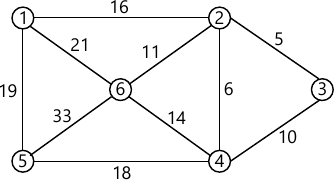MORE IN Data Structures & Algorithms
SPPU Electronics and Telecom Engineering (Semester 3)
Data Structures & Algorithms
June 2015
Total marks: --
Total time: --
INSTRUCTIONS
(1) Assume appropriate data and state your reasons
(2) Marks are given to the right of every question
(3) Draw neat diagrams wherever necessary

Answer any one question from Q1 and Q2
1 (a) What do you mean by recursive function? Explain with example.
6 M
1 (b) Write a C function for insertion sort to sort integer numbers.
6 M

2 (a) Explain parameter passing by value and passing parameter by reference with suitable example.
6 M
2 (b) What is pointer ? What are the advantages of using pointer? Explain pointer declaration and its initialization with an example.
6 M

Answer any one question from Q3 and Q4
3 (a) What is singly linked list ? Write C function for inserting a node at a given location into a Singly Linked List.
6 M
3 (b) Evaluate the following postfix expression using stack
623+ -382/+*2∧.
Note: ∧ stands for power and all operands are single digit.
7 M

4 (a) Write short notes on:
6 M
4 (b) What is priority queue? Explain its implementation using any one method.
7 M

Answer any one question from Q5 and Q6
5 (a) What is Binary Search Tree (BST)? Write C functions for:
(i) Finding the smallest number in BST
(ii) Recursive inorder traversal of BST.
7 M
5 (b) What is AVL Tree ? Define balance factor. Explain RR rotation with an example.
5 M

6 (a) What is Binary Search Tree (BST)? Construct a BST for the following numbers:
27, 42, 43, 17, 39, 31, 10, 9, 19, 54, 33, 48.
Show all the steps. Write its preorder traversal
8 M
6 (b) Explain threaded binary tree with an example. What is its advantage?
4 M

Answer any one question from Q7 and Q8
7 (a) Write C function to implement Depth First Search traversal of a graph implemented using adjacency matrix.
6 M
7 (b) What do you mean by indegree and outdegree of a vertex in a graph ? Write a C function to find indegree and outdegree of vertex in a graph implemented using adjacency matrix.
7 M

8 (a) Define the term Graph. With the help of suitable example give adjacency matrix representation and adjacency list representation of a graph.
7 M
8 (b) What do you mean by spanning tree of a graph ? Find the minimal spanning tree of the following graph using Kruskal's algorithm. (Refer Fig. 1).6 M

More question papers from Data Structures & Algorithms